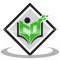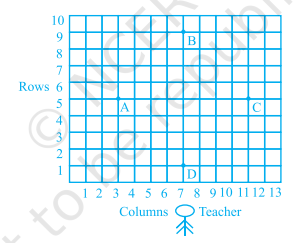## If $\cos 9 \alpha=\sin \alpha$ and $9 \alpha<90^{\circ}$, then the value of $\tan 5 \alpha$ is(A) $\frac{1}{\sqrt{3}}$(B) $\sqrt{3}$(C) 1(D) 0Updated on 10-Oct-2022 13:28:52
Given:$\cos 9 \alpha=\sin \alpha$ and $9 \alpha ## The value of \( \left(\tan 1^{\circ} \tan 2^{\circ} \tan 3^{\circ} \ldots \tan 89^{\circ}\right)$ is(A) 0(B) 1(C) 2(D) $\frac{1}{2}$Updated on 10-Oct-2022 13:28:52
Given:$\left(\tan 1^{\circ} \tan 2^{\circ} \tan 3^{\circ} \ldots \tan 89^{\circ}\right)$To do:We have to find the value of $\left(\tan 1^{\circ} \tan 2^{\circ} \tan 3^{\circ} \ldots \tan 89^{\circ}\right)$.Solution:  We know that, $tan\ (90^{\circ}- \theta) = cot\ \theta$$tan\ \theta \times \cot\ \theta=1Therefore, \tan 1^{\circ} \tan 2^{\circ} \tan 3^{\circ}.....\tan 45^{\circ}...... \tan 87^{\circ} \tan 88^{\circ} \tan 89^{\circ}=\tan 1^{\circ} \tan 2^{\circ} \tan 3^{\circ}......\tan 45^{\circ}.........\tan (90^{\circ}-3^{\circ}) \tan (90^{\circ}-2^{\circ}) \tan (90^{\circ}-1^{\circ})$$=\tan 1^{\circ} \tan 2^{\circ} \tan 3^{\circ}........\tan 45^{\circ}......\cot 3^{\circ} \cot 2^{\circ} \cot 1^{\circ}$$=(\tan 1^{\circ} \cot 1^{\circ})(\tan 2^{\circ} \cot 2^{\circ})..................(\tan 44^{\circ} \cot 44^{\circ})(1)$$=1$Read More

## If $\cos (\alpha+\beta)=0$, then $\sin (\alpha-\beta)$ can be reduced to(A) $\cos \beta$(B) $\cos 2 \beta$(C) $\sin \alpha$(D) $\sin 2 \alpha$Updated on 10-Oct-2022 13:28:52

## Students of a school are standing in rows and columns in their playground for a drill practice. $\mathrm{A}, \mathrm{B}, \mathrm{C}$ and $\mathrm{D}$ are the positions of four students as shown in figure below. Is it possible to place Jaspal in the drill in such a way that he is equidistant from each of the four students $A, B, C$ and D? If so, what should be his position?"Updated on 10-Oct-2022 13:28:51
Given:Students of a school are standing in rows and columns in their playground for a drill practice. $\mathrm{A}, \mathrm{B}, \mathrm{C}$ and $\mathrm{D}$ are the positions of four students.To do:We have to find whether it is possible to place Jaspal in the drill in such a way that he is equidistant from each of the four students $A, B, C$ and D.Solution:From the figure, we observe that the positions of the four students A, B, C and D are $(3, 5), (7, 9), (11, 5)$ and $(7, 1)$ respectively.The four vertices form a quadrilateral. $A B ... Read More ## If the poins $A(1,-2), B(2,3) C(a, 2)$ and $D(-4,-3)$ form a parallelogram. find the value of $a$ and height of the parallelogram taking AB as base.Updated on 10-Oct-2022 13:28:51 Given:The points$A (1, -2), B (2, 3), C (a, 2)$and$D (-4, -3)$form a parallelogram.To do:We have to find the value of$a$and height of the parallelogram taking$AB$as base.Solution:Draw a perpendicular from $\mathrm{D}$ to $\mathrm{AB}$ which meets $\mathrm{AB}$ at $\mathrm{P}$.$\mathrm{DP}$ is the height of the parallelogram. We know that, Diagonals of a parallelogram bisect each other.This implies, Mid-point of$AC =$Mid-point of$BD$The mid-point of a line segment joining points $\left(x_{1}, y_{1}\right)$ and $\left(x_{2}, y_{2}\right)$ is $(\frac{x_{1}+x_{2}}{2}, \frac{y_{1}+y_{2}}{2})$$(\frac{1+a}{2}, ... Read More ## The points \( A\left(x_{1}, y_{1}\right), \mathrm{B}\left(x_{2}, y_{2}\right)$ and $\mathrm{C}\left(x_{3}, y_{3}\right)$ are the vertices of $\Delta \mathrm{ABC}$What are the coordinates of the centroid of the triangle ABC?Updated on 10-Oct-2022 13:28:51 Given:The points $A\left(x_{1}, y_{1}\right), \mathrm{B}\left(x_{2}, y_{2}\right)$ and $\mathrm{C}\left(x_{3}, y_{3}\right)$ are the vertices of $\Delta \mathrm{ABC}$To do:We have to find the coordinates of the centroid of the triangle ABC.Solution:We know that,Coordinates of the centroid of a triangle$=\left(\frac{\text { Sum of abscissa of all vertices, }}{3}, \frac{\text { Sum of ordinate of all vertices }}{3}\right)$Therefore,The coordinates of the centroid of the triangle ABC$=\left(\frac{x_{1}+x_{2}+x_{3}}{3}, \frac{y_{1}+y_{2}+y_{3}}{3}\right)\$
Previous 1 ... 5 6 7 8 9 ... 840 Next